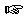### Section 5

Problem 1:
Develop the function describe-temp, which consumes a Celsius temperature and returns 'Warm if the temperature is 25 or greater, 'Mild if the temperature is between 10 and 25, and 'Cold if the temperature is below 10.Solution

Problem 2:
Develop a function that when given a symbol and number returns the square root of the number if the symbol is 'squareroot or the tangent of the number if the symbol is 'tangent. For all other symbols, it returns the original number.Solution

Problem 3:
Modify check-guess from Exercise 5.1.2 (duplicated below) to add a fourth response, 'WithinTen, which is returned when the player's guess is in the range of ten above or below the target.

Exercise 5.1.2

Develop the function check-guess. It consumes two numbers, guess and target. Depending on how guess relates to target, the function produces one of the following three answers: 'TooSmall, 'Perfect, or 'TooLarge.

The function implements one part of a two-player number guessing game. One player picks a random number between 0 and 99999. The other player's goal is to determine this number, called target, with the least number of guesses. To each guess, the first player responds with one of the three responses that check-guess implements.

The function check-guess and the teachpack guess.ss implement the first player. The teachpack picks the random number, pops up a window in which the second player can choose digits, and hands over the guess and the target to check-guess. To play the game, set the teachpack to guess.ss using the `Language|Set teachpack` option. Then evaluate the expression

```(guess-with-gui check-guess)
```
after check-guess has been thoroughly tested.Solution

Problem 4:

Inhabitants of the island of knaves always walk around in pairs. Each will respond with 'Yes or 'No individually when asked an appropriate question. You can only be certain of having received an affirmative answer if both reply 'Yes. All other combinations imply 'No.

Develop the function discern, which will accept all pairwise combinations of knaves' answers and produce 'Yes or 'No accordingly.Solution

Problem 5:

A robot car is confined to a narrow straight track that is marked with positions 0 through 99.

Develop the function move. It consumes a command (either 'Forward or 'Backward), the number of units to move, and the current position of the robot, then returns the new position of the robot. The robot cannot go off the end of the track, so once it has reached either end it will remain there.Solution

 Jamie Raymond Matthias Felleisen 01 december 2003